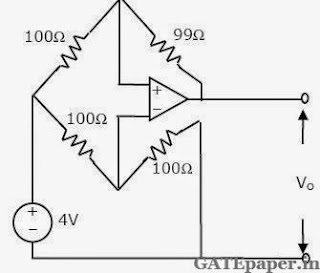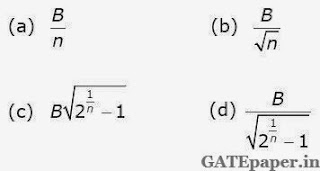### GATE 1993 ECE Analog Circuits (Analog Electronics) - Video Solutions

1. For the amplifier circuit of figure, the transistor has a β of 800. The mid band voltage gain Vo/V1 of the circuit will bea. 0
b. < 1
c. = 1
d. 800

2. For the ideal operational amplifier circuit shown, determine the output voltage Vo.3. α – cutoff frequency of a bipolar junction transistor
a. increases with the increase in base width
b. increases with the increase in emitter width
c. increase with increase in the collector width
d. increase with decrease in the base width

4. Negative feedback in amplifiers
a. improves the signal to noise ratio at the input
b. improves the signal to noise ratio at the output
c. does not affect the signal to noise ratio at the output
d. reduces distortion

5. In a multi stage RC coupled amplifier, the coupling capacitor
a. limits the low frequency response
b. limits the high frequency response
c. does not affect the frequency response
d. blocks the DC component without affecting the frequency response

6. The bandwidth of an n-stage tuned amplifier, with each stage having a bandwidth of B, is given by7. In a transistor push-pull amplifier
a. there is no DC present at the output
b. there is no distortion in the output
c. there are no even harmonics in the output
d. there are no odd harmonics in the output

8. A JFET with the following parameters is used in a single stage common source amplifier with a load resistance of 100 kΩ. Calculate the high frequency cutoff (upper 3dB frequency) of the amplifier. Given gm = 2 mA/V, Cgd = 2 pF, Cds = 2 pF, rd = 100 kΩ and Cgs = 1 pF.

9. Find the output voltage Vo in the following circuit, assuming that the operational amplifier is ideal.